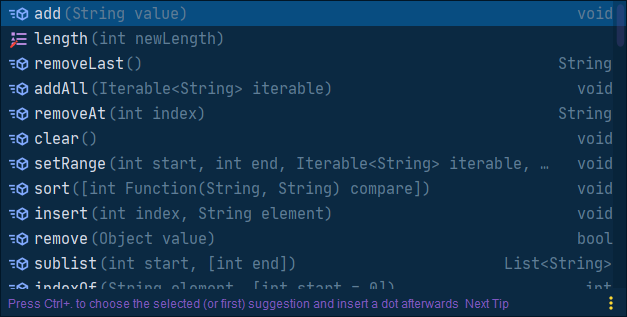Stars-oneAndroid Kotlin Java开发者,喜欢折腾搞机,开发小工具

• 累计撰写 211 篇文章
• 累计创建 45 个标签
• 累计收到 23 条评论

### 目 录CONTENT# Flutter学习(2)——Dart语言快速入门2020-08-28 / 0 评论 / 0 点赞 / 604 阅读 / 0 字

Dart语言的语法和Kotlin、Java有类似之处，这里就通过对比Java和Kotlin来快速入门Dart语言

## 1.变量与类型

### 1.1 num类型

int b1;
print(b1);//输出为null


Dart特有整除运算符~/,两个int相除并不是得到int类型的数值,而是double类型

print(300 / 100);//输出为3.0
print(6 ~/ 5 );//输出为1


num类型常用的方法:

main() {
print(3.141592653.toStringAsFixed(3)); //3.142 保留有效数字
print(6.6.floor());//6向下取整
print((-6.6).ceil()); //-6 向上取整
print(9.9.ceil()); //10 向上取整
print(666.6.round()); //667 四舍五入
print((-666.6).abs()); // 666.6 取绝对值
print(666.6.toInt()); //666 转化成int,这中toInt、toDouble和Kotlin类似
print(999.isEven); //false 是否是偶数
print(999.isOdd); //true 是否是奇数
print(666.6.toString()); //666.6 转化成字符串
}


String类型的特性和Kotlin差不多,支持单引号、双引号、三引号以及$字符串模板用法 ### 1.3 Object与Dynamic 和Java一样,Object是所有对象的父类,而Dynamic是一种特殊的类型,主要用于不确定的类型 ### 1.4 类型判断/转换 Dart语言与Kotlin一样，具有类型推导功能 如: var b = true;//b变量的类型为bool  类型检查(is和is!)和强制类型转换(as) main() { int number = 100; double distance = 200.5; num age = 18; print(number is num);//true print(distance is! int);//true print(age as int);//18 }  ### 1.5 变量和常量 Dart中,变量可以使用var关键字,会自动的进行类型推导,如果在使用var定义了一个变量,并没有初始化赋值,这个变量的类型是会随着赋值的类型而改变(dynamic类型的特点) void main() { var num; num = 123; print(num is int);/true num ="xx"; print(num is String);//true var t = "xx"; t = 123; //IDE会提示错误 }  constfinal都是定义常量的关键字,const编译期就能初始化(相当于Kotlin中的final val,而final运行期初始化(相当于Kotlin中的val) ## 2.方法/函数 这里方法其实与Java中非常类似,这里就提及Dart中的方法比较特殊几点 Dart中的方法格式和Java中十分类似,基本格式为 返回值 方法名(参数类型 参数名...)  值得一提的是,返回值并不是必须的(JavaScript的语言特性?),你可以省略不写,但是仍可以使用return返回数值,建议实际开发中不要这样写,你懂得... 当然,如果返回值为空,你可以省事不写或者是定义返回值为void void main{ ... } //与上面的相同 main{ ... }  Dart中可以使用箭头函数,与Java和Kotlin中的lambda方式->很像 main() => print("hello world");  比较特殊的是,Dart中支持参数默认赋值以及命名参数(Kotlin中也有),然后值得一提的是,Dart中并不支持函数重载操作,包括构造函数 普通方法定义: num sum(int a,int b){ print(a+b); }  特殊定义: num sum({int a,int b}){ print(a); print(b); }  个人感觉好像是使用了Json对象作为了参数类型,之后调用的时候参数可选,但是得标明参数名 main{ sum(a:1); sum(b:2); sum(1,2);//IDE会报错 sum(a:1,b:2); }  上面说了,{}可以看做为是一种Json类型,所以,我们可以将其和普通的类型组合起来构造参数列表 num sum(int c,{int a,int b}){ print(a); print(b); }  PS:我不知道我这样称呼{}为Json类型对不对,只是单纯觉得好理解... ## 3.类、接口、抽象类 ### 3.1 类 与Java和Kotlin一样，Dart也是通过class关键字来定义个类 class Person { var name; //隐藏了默认的无参构造函数Person(); } //等价于: class Person { var name; Person();//一般把与类名相同的函数称为主构造函数 } //等价于 class Person { var name; Person(){} } class Person { final String name; final int age; Person(this.name, this.age);//显式声明有参主构造函数 Person();//编译异常，注意: dart不支持同时重载多个构造函数。 }  在之前提及了构造函数不能被重载的概念,但实际很多情况都是要根据不同的参数列表来实例化一个类对象,不过好在Dart有个命名构造函数的概念,我们可以利用它来实现曲线救国。 class Person { final String name; int age; Person(this.name, this.age); Person.withName(this.name);//通过类名.函数名形式来定义命名构造函数withName。只需要name参数就能创建对象， //如果没有命名构造函数，在其他语言中，我们一般使用函数重载的方式实现。 } main () { var person = Person('mikyou', 18);//通过主构造函数创建对象 var personWithName = Person.withName('mikyou');//通过命名构造函数创建对象 }  重定向构造函数: 有时候我们定义了个参数列表比较全的构造方法,并且某些参数是具有默认值,使用的时候可以省略某些参数,这种情况下,我们就可以使用重定向构造函数来达到我们的效果,我们使用:来实现,如: class Person{ String name; int age; Person(this.name,this.age); Person.withName(String name):this(name,18); } main{ var person = Person.withName("小红");//默认小红为18岁 }  Dart中的继承也是使用extends关键字 class Person{ String name; int age; //含有默认的构造函数 } //继承 class Student extends Person{ }  ### 3.2 接口 需要注意的是，Dart中并没有接口对应的关键字interface，如果我们想要实现某个接口,得将某个类声明为抽象类,并定义好接口方法,之后通过implements关键字去实现该类的接口方法即可(有点类似实现抽象类的感觉) abstract class OnClickListener{ void onClick(); } class View implements OnClickListener{ @override void onClick() { } }  ## 4.集合 ### 4.1 List Dart中的List和数组有点像,List的初始化方式如下所示: List<String> colorList = ['red', 'yellow', 'blue', 'green'];//直接使用[]形式初始化 var colorList = <String> ['red', 'yellow', 'blue', 'green'];  常用的方法其实挺多的,打个.就可以看到了,这里也就不多提了遍历方法: main() { List<String> colorList = ['red', 'yellow', 'blue', 'green'];//for-i遍历 for(var i = 0; i < colorList.length; i++) {//可以使用var或int print(colorList[i]); } //forEach遍历 colorList.forEach((color) => print(color));//forEach的参数为Function. =>使用了箭头函数 //for-in遍历 for(var color in colorList) { print(color); } //while+iterator迭代器遍历，类似Java中的iteator while(colorList.iterator.moveNext()) { print(colorList.iterator.current); } }  ### 4.2 Set 集合Set和列表List的区别在于 集合中的元素是不能重复 的。所以添加重复的元素时会返回false,表示添加不成功. main() { Set<String> colorSet= {'red', 'yellow', 'blue', 'green'};//直接使用{}形式初始化 var colorList = <String> {'red', 'yellow', 'blue', 'green'}; }  Dart中可使用交、并、补集: main() { var colorSet1 = {'red', 'yellow', 'blue', 'green'}; var colorSet2 = {'black', 'yellow', 'blue', 'green', 'white'}; print(colorSet1.intersection(colorSet2));//交集-->输出: {'yellow', 'blue', 'green'} print(colorSet1.union(colorSet2));//并集--->输出: {'black', 'red', 'yellow', 'blue', 'green', 'white'} print(colorSet1.difference(colorSet2));//补集--->输出: {'red'} }  遍历方式与List一样,这里不多提 ### 4.3 Map 初始化方式: main() { Map<String, int> colorMap = {'white': 0xffffffff, 'black':0xff000000};//使用{key:value}形式初始化 var colorMap = <String, int>{'white': 0xffffffff, 'black':0xff000000}; }  遍历: main() { Map<String, int> colorMap = {'white': 0xffffffff, 'black':0xff000000}; //for-each key-value colorMap.forEach((key, value) => print('color is$key, color value is $value')); }  List转为Map: main() { List<String> colorKeys = ['white', 'black']; List<int> colorValues = [0xffffffff, 0xff000000]; Map<String, int> colorMap = Map.fromIterables(colorKeys, colorValues); }  ### 4.4 集合常用操作符 main() { List<String> colorList = ['red', 'yellow', 'blue', 'green']; //forEach箭头函数遍历 colorList.forEach((color) => {print(color)}); colorList.forEach((color) => print(color)); //箭头函数遍历，如果箭头函数内部只有一个表达式可以省略大括号 //map函数的使用,输出以,分隔的字符串 print(colorList.map((color) => '$color').join(","));

//every函数的使用，判断里面的元素是否都满足条件，返回值为true/false
print(colorList.every((color) => color == 'red'));

//sort函数的使用
List<int> numbers = [0, 3, 1, 2, 7, 12, 2, 4];
numbers.sort((num1, num2) => num1 - num2); //升序排序
numbers.sort((num1, num2) => num2 - num1); //降序排序
print(numbers);

//where函数使用，相当于Kotlin中的filter操作符，返回符合条件元素的集合
print(numbers.where((num) => num > 6));

//firstWhere函数的使用，相当于Kotlin中的find操作符，返回符合条件的第一个元素，如果没找到返回null
print(numbers.firstWhere((num) => num == 5, orElse: () => -1)); //注意: 如果没有找到，执行orElse代码块，可返回一个指定的默认值

//singleWhere函数的使用，返回符合条件的第一个元素，如果没找到返回null，但是前提是集合中只有一个符合条件的元素, 否则就会抛出异常
print(numbers.singleWhere((num) => num == 4, orElse: () => -1)); //注意: 如果没有找到，执行orElse代码块，可返回一个指定的默认值

//take(n)、skip(n)函数的使用，take(n)表示取当前集合前n个元素, skip(n)表示跳过前n个元素，然后取剩余所有的元素
print(numbers.take(5).skip(2));

//List.from函数的使用，从给定集合中创建一个新的集合,相当于clone一个集合
print(List.from(numbers));

//expand函数的使用, 将集合一个元素扩展成多个元素或者将多个元素组成二维数组展开成平铺一个一位数组
var pair = [
[1, 2],
[3, 4]
];
print('flatten list: ${pair.expand((pair) => pair)}'); var inputs = [1, 2, 3]; print('duplicated list:${inputs.expand((number) =>[
number,
number,
number
])}');
}


## 5.总结

1. 函数不能重载
2. 构造函数不能重载,但可以用命名构造函数来变相实现重载的功能
3. 方法返回值可以省略,但仍可以使用return返回数值(JS特性)
4. 函数参数有个特殊的类型{}(Json类型?)
5. Dart中没有接口,需要使用抽象类来实现接口

0